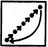# Non Verbal Reasoning - Series

### Exercise :: Series - Section 1

Each of the following questions consists of five figures marked A, B, C, D and E called the Problem Figures followed by five other figures marked 1, 2, 3, 4 and 5 called the Answer Figures. Select a figure from amongst the Answer Figures which will continue the same series as established by the five Problem Figures.

6.

Select a figure from amongst the Answer Figures which will continue the same series as established by the five Problem Figures.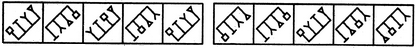(A)     (B)     (C)     (D)     (E)       (1)     (2)     (3)     (4)     (5)

 A. 1 B. 2 C. 3 D. 4 E. 5

Explanation:

Similar figure reappears in every second step. Each time the first figure reappears, the elements interchange positions in the order :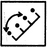. And, each time the second figure reappears, the elements interchange positions in the order :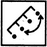7.

Select a figure from amongst the Answer Figures which will continue the same series as established by the five Problem Figures.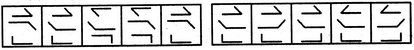(A)     (B)     (C)     (D)     (E)       (1)     (2)     (3)     (4)     (5)

 A. 1 B. 2 C. 3 D. 4 E. 5

Explanation:

In One step, the middle element rotates through 180o and in the next step, the other two elements rotate through 180o. The two steps are repeated alternately.

8.

Select a figure from amongst the Answer Figures which will continue the same series as established by the five Problem Figures.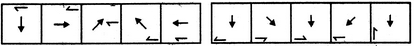(A)     (B)     (C)     (D)     (E)       (1)     (2)     (3)     (4)     (5)

 A. 1 B. 2 C. 3 D. 4 E. 5

Explanation:

The central arrow rotates 90oACW and 45oCW alternately. The half-arrow moves half-a-side of the square boundary in a CW direction and its head turns to the other side of the line in each step.

9.

Select a figure from amongst the Answer Figures which will continue the same series as established by the five Problem Figures.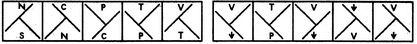(A)     (B)     (C)     (D)     (E)       (1)     (2)     (3)     (4)     (5)

 A. 1 B. 2 C. 3 D. 4 E. 5

Explanation:

The 'T' shaped large element rotates 90oCW in each step. The two small symbols interchange positions and the symbol that reaches the upper position gets replaced by a new one.

10.

Select a figure from amongst the Answer Figures which will continue the same series as established by the five Problem Figures.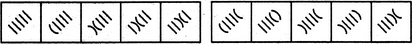(A)     (B)     (C)     (D)     (E)       (1)     (2)     (3)     (4)     (5)

 A. 1 B. 2 C. 3 D. 4 E. 5

In the first step, the lowermost line segment is converted into a curve. In the second step, the second line segment also gets converted into a curve and the (existing curve is inverted. In each subsequent step, all the elements (line segments and curves) move in the sequence